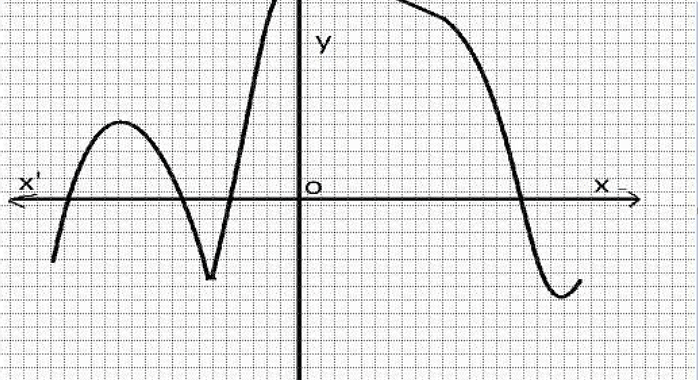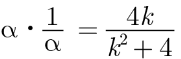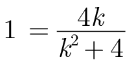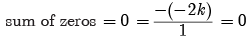Test: Polynomials- Assertion & Reason Type Questions- 1

# Test: Polynomials- Assertion & Reason Type Questions- 1 - Class 8

Test Description

## 10 Questions MCQ Test Advance Learner Course: Mathematics (Maths) Class 8 - Test: Polynomials- Assertion & Reason Type Questions- 1

Test: Polynomials- Assertion & Reason Type Questions- 1 for Class 8 2023 is part of Advance Learner Course: Mathematics (Maths) Class 8 preparation. The Test: Polynomials- Assertion & Reason Type Questions- 1 questions and answers have been prepared according to the Class 8 exam syllabus.The Test: Polynomials- Assertion & Reason Type Questions- 1 MCQs are made for Class 8 2023 Exam. Find important definitions, questions, notes, meanings, examples, exercises, MCQs and online tests for Test: Polynomials- Assertion & Reason Type Questions- 1 below.
Solutions of Test: Polynomials- Assertion & Reason Type Questions- 1 questions in English are available as part of our Advance Learner Course: Mathematics (Maths) Class 8 for Class 8 & Test: Polynomials- Assertion & Reason Type Questions- 1 solutions in Hindi for Advance Learner Course: Mathematics (Maths) Class 8 course. Download more important topics, notes, lectures and mock test series for Class 8 Exam by signing up for free. Attempt Test: Polynomials- Assertion & Reason Type Questions- 1 | 10 questions in 20 minutes | Mock test for Class 8 preparation | Free important questions MCQ to study Advance Learner Course: Mathematics (Maths) Class 8 for Class 8 Exam | Download free PDF with solutions
 1 Crore+ students have signed up on EduRev. Have you?
Test: Polynomials- Assertion & Reason Type Questions- 1 - Question 1

### Direction: In the following questions, a statement of assertion (A) is followed by a statement of reason (R). Mark the correct choice as:Assertion : (2 - √3) is one zero of the quadratic polynomial then other zero will be (2 + √3)Reason : Irrational zeros (roots) always occurs in

Detailed Solution for Test: Polynomials- Assertion & Reason Type Questions- 1 - Question 1
As irrational roots/zeros always occurs in pairs therefore, when one zero is (2 - √3) then other will be 2 + √3 . So, both A and R are correct and R explains A.

Thus (a) is correct option.

Test: Polynomials- Assertion & Reason Type Questions- 1 - Question 2

### Direction: In the following questions, a statement of assertion (A) is followed by a statement of reason (R). Mark the correct choice as:Assertion : The graph y = f(x) is shown in figure, for the polynomial f(x). The number of zeros of f(x) is 4. Reason : The number of zero of the polynomial f(x). is the number of point of which f(x) cuts or touches the axes.

Detailed Solution for Test: Polynomials- Assertion & Reason Type Questions- 1 - Question 2

As the number zero of polynomial f(x) is the number of points at which f(x) cuts (intersects) the x -axis and number of zero in the given figure is 4. So A is correct but R is incorrect.

Test: Polynomials- Assertion & Reason Type Questions- 1 - Question 3

### Direction: In the following questions, a statement of assertion (A) is followed by a statement of reason (R). Mark the correct choice as:Assertion : If p(x) ax + b, a ≠ 0 is a linear polynomial, then x = -b/a is the only zero of p(x).Reason : A linear polynomial has one and only one zero.

Detailed Solution for Test: Polynomials- Assertion & Reason Type Questions- 1 - Question 3
When a curve or a line intersects X-axis then the y-co ordinate will be zero.

Because on X-axis the value of y-co ordinate is zero.

Given equation is y = ax + b

Now on substituting y = 0 we get ax + b = 0

⇒ ax = −b

⇒ x = -b/a​

Therefore the co-ordinates are ( -b/a, 0).

Test: Polynomials- Assertion & Reason Type Questions- 1 - Question 4

Direction: In the following questions, a statement of assertion (A) is followed by a statement of reason (R). Mark the correct choice as:

Assertion : If one zero of poly-nominal p(x) = (k2 + 4) x2 + 13x + 4k is reciprocal of other,

then k = 2.

Reason : If (x - α) is a factor of p(x), then p(α) = 0 i.e. α is a zero of p(x).

Detailed Solution for Test: Polynomials- Assertion & Reason Type Questions- 1 - Question 4
Let α, 1/α be the zeros of p(x), thenK2 - 4k + 4 = 0

(k - 2)2 = 0 ⇒ k = 2

Assertion is true Since, Reason is not correct for Assertion.

Thus (b) is correct option.

Test: Polynomials- Assertion & Reason Type Questions- 1 - Question 5

Direction: In the following questions, a statement of assertion (A) is followed by a statement of reason (R). Mark the correct choice as:

Assertion : Degree of a zero polynomial is not defined.

Reason : Degree of a non-zero constant polynomial is ‘0’

Detailed Solution for Test: Polynomials- Assertion & Reason Type Questions- 1 - Question 5
We know that,

The constant polynomial 0 is called a zero polynomial.

The degree of a zero polynomial is not defined.

∴ Assertion is true.

The degree of a non-zero constant polynomial is zero.

∴ Reason is true.

Since both Assertion and Reason are true and Reason is not a correct explanation of Assertion.

Test: Polynomials- Assertion & Reason Type Questions- 1 - Question 6

Direction: In the following questions, a statement of assertion (A) is followed by a statement of reason (R). Mark the correct choice as:

Assertion : The degree of the polynomial (x - 2) (x- 3) (x + 4) is 4.

Reason : The number of zeroes of a polynomial is the degree of that polynomial.

Detailed Solution for Test: Polynomials- Assertion & Reason Type Questions- 1 - Question 6
p(x) = (x - 2)(x - 3)(x + 4)

= (x - 2)[x2 + 4x - 3x - 12]

= (x - 2)(x2 + x - 12)

= x3 + x2 - 12x - 2x2 - 2x + 24

p(x) = x3 - x2 - 14x + 24

So, degree of p(x) h = 3

Test: Polynomials- Assertion & Reason Type Questions- 1 - Question 7

Direction: In the following questions, a statement of assertion (A) is followed by a statement of reason (R). Mark the correct choice as:

Assertion : p(x) = 14x3 - 2x2 + 8x4 + 7x - 8 is a polynomial of degree 3.

Reason : The highest power of x in the polynomial p(x) is the degree of the polynomial.

Detailed Solution for Test: Polynomials- Assertion & Reason Type Questions- 1 - Question 7
The highest power of x in the polynomial p(x) = 14x3 - 2x2 + 8x4 + 7x - 8 is 4. Degree of p(x) is 4. So, A is incorrect but R is correct.

Thus (d) is correct option.

Test: Polynomials- Assertion & Reason Type Questions- 1 - Question 8

Direction: In the following questions, a statement of assertion (A) is followed by a statement of reason (R). Mark the correct choice as:

Assertion : If both zeros of the quadratic polynomial x2 - 2kx + 2 are equal in magnitude but opposite in sign then value of k is 1/2.

Reason : Sum of zeros of a quadratic polynomial ax2 + bx + c is -b/a

Detailed Solution for Test: Polynomials- Assertion & Reason Type Questions- 1 - Question 8
As the polynomial is x2 - 2kx + 2 and its zeros are equal but opposition sign⇒ 2k = 0 & k = 0

So, A is incorrect but R is correct.

Test: Polynomials- Assertion & Reason Type Questions- 1 - Question 9

Direction: In the following questions, a statement of assertion (A) is followed by a statement of reason (R). Mark the correct choice as:

Assertion : If f(x) = x4 + x3 - 2x2 + x + 1 is divided by (x - 1), then its remainder is 2.

Reason : If p(x) be a polynomial of degree greater than or equal to one, divided by the linear polynomial x - a , then the remainder is p(-a).

Detailed Solution for Test: Polynomials- Assertion & Reason Type Questions- 1 - Question 9
According to remainder theorem: Let p(x) be any polynomial of degree greater than or equal to one and is divided by the linear polynomial x - a where a be any number which would be the divisor and we get the value of x = a, then the remainder is p (-a). Factor theorem is a special case for the remainder theorem.
Test: Polynomials- Assertion & Reason Type Questions- 1 - Question 10

Direction: In the following questions, a statement of assertion (A) is followed by a statement of reason (R). Mark the correct choice as:

Assertion : x3 + x has only one real zero.

Reason : A polynomial of n th degree must have n real zeroes.

Detailed Solution for Test: Polynomials- Assertion & Reason Type Questions- 1 - Question 10
A polynomial of nth degree at most can have n real zeroes. Thus reason is not true.

Again, x3 + x = x(x2 + 1)

which has only one real zero because x2 + 1 ≠ 0 for all x ∈ R.

Assertion (A) is true but reason (R) is false.

Thus (c) is correct option.

## Advance Learner Course: Mathematics (Maths) Class 8

13 videos|62 docs|24 tests
Information about Test: Polynomials- Assertion & Reason Type Questions- 1 Page
In this test you can find the Exam questions for Test: Polynomials- Assertion & Reason Type Questions- 1 solved & explained in the simplest way possible. Besides giving Questions and answers for Test: Polynomials- Assertion & Reason Type Questions- 1, EduRev gives you an ample number of Online tests for practice

## Advance Learner Course: Mathematics (Maths) Class 8

13 videos|62 docs|24 tests